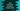# Java program to print below and above average marks students## Java program to print below and above average marks students :

In this Java programming tutorial, we will learn how to find the students who got above or below average marks from a list of students. Mainly, our program will create a list of students with different name and marks for each student. It will then find out the average marks of all of these students. Finally, it will print out the students whose marks is below average and above average.

This program will teach you how to use a for-loop, how to create objects of custom class, and how to use ArrayList in java.

Let’s take a look at the program first :

``````import java.util.ArrayList;
import java.util.List;

class Main {
public static void main(String[] args) {
//1
List studentList = new ArrayList<>();

//2
Student Alex = new Student("Alex", 24);
Student Albert = new Student("Albert", 74);
Student Brook = new Student("Brook", 47);
Student Carlos = new Student("Carlos", 71);
Student Ira = new Student("Ira", 84);
Student Jill = new Student("Jill", 61);
Student Lucy = new Student("Lucy", 22);
Student Nancy = new Student("Nancy", 94);

//3

//4
float avgNo = findAverage(studentList);

//6
for (int i = 0; i < studentList.size(); i++) {
if (studentList.get(i).getMarks() < avgNo) { System.out.println(studentList.get(i).getName() + " got " + studentList.get(i).getMarks() + " ---> Below Average ");
} else {
System.out.println(studentList.get(i).getName() + " got " + studentList.get(i).getMarks() + " ---> Above Average ");
}
}
}

//5
private static float findAverage(List list) {
float sum = 0;

for (int i = 0; i < list.size(); i++) {
sum += list.get(i).getMarks();
}

return sum / list.size();
}
}

class Student {
private String name;
private int marks;

public Student(String name, int marks) {
this.name = name;
this.marks = marks;
}

public String getName() {
return name;
}

public int getMarks() {
return marks;
}
}``````

### Explanation :

The commented numbers in the above program denote the step number below :

1. Create one ArrayList studentList to hold different Student object. Objects of type Student can hold two parameters : one string variable name and one int marks.
2. Create different Student objects with different name and marks. In this example, we are creating 8 different Student objects.
3. Add these objects to the ArrayList studentList.
4. Now, find out the average numbers of these Student objects. We have findAverage method to find out the average marks.
5. findAverage method takes one arraylist as parameter. It calculates the average marks of all Student in the arraylist and returns this value. The average marks is a floating value.
6. To find the students with marks below average and above average, scan each element of studentList one by one. Compare the marks of each Student of the list with the average value and print out the result.

### Output :

The above program will print out the below output :

``````Alex got 24 ---> Below Average
Albert got 74 ---> Above Average
Brook got 47 ---> Below Average
Carlos got 71 ---> Above Average
Ira got 84 ---> Above Average
Jill got 61 ---> Above Average
Lucy got 22 ---> Below Average
Nancy got 94 ---> Above Average ``````

You can also try to modify the program and add different types of objects to the list. Try to add more Student to the ArrayList and drop one comment below if you have any queries.